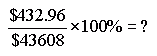From: Cheryl Kiera invested \$43,608 in a mutual fund that paid \$432.96 in interest at the end of the year.  What was the percent of interest Kiera earned from her initial investment? I need to know how to come up with the answer of 1%. Hi Cheryl. A percentage is just a ratio - which means fraction. If you divide \$432.96 into \$43 608 and then multiply by one hundred, you will get the percentage interest Kiera earned.Hope this helps, Sue